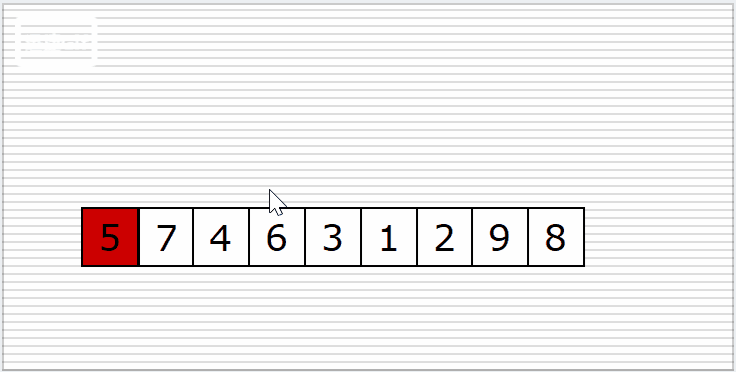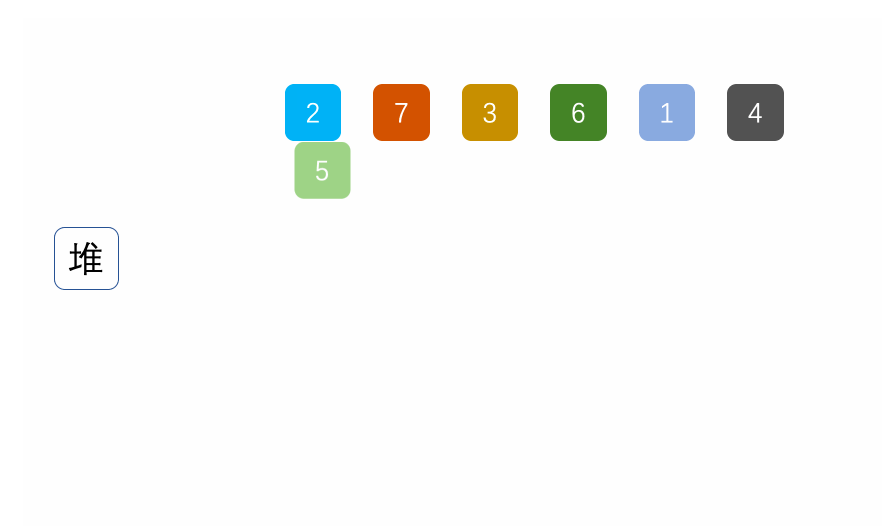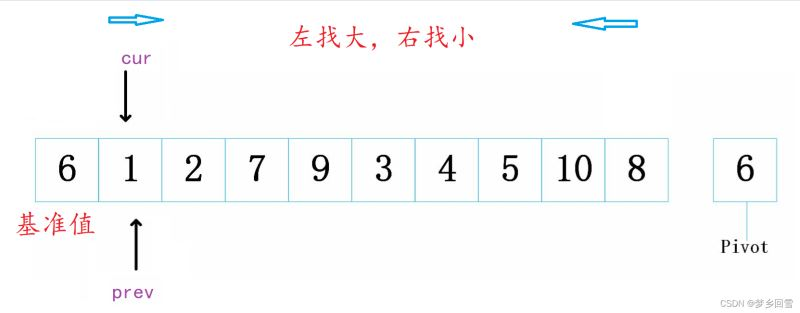﻿ C语言 八大排序算法的过程图解及实现代码_C 语言_脚本之家
C 语言# C语言 八大排序算法的过程图解及实现代码

## 前言

• 排序稳定性：多个具有相同的关键字的记录，若经过排序，这些记录的相对次
• 序保持不变，即在原序列中，r[i]=r[j]，且r[i]在r[j]之前，而在排序后的序列中，r[i]仍在r[j]之前，则称这种排序算法是稳定的；否则称为不稳定的。
• 内部排序：数据元素全部放在内存中的排序。
• 外部排序：数据元素太多不能同时放在内存中，根据排序过程的要求不能在内外存之间移动数据的排序。

## 一、插入排序

### 空间复杂度

O(1)

-『 插入排序 』：顾名思义就是把每一个数插入到有序数组中对应的位置。### 代码实现（升序）

```void InsertSort(int* a, int n)
{
for (int i = 0; i < n - 1; i++)
{
int x = a[end+1];//x为待排序的值
int end = i;//从end开始往前和x依次比较

while (end >= 0)
{
if (a[end] > x)//只要当前的值大于x继续往前找
{
a[end+1] = a[end];
end--;
}
else
{
break;//跳出循环说明a[end] <= x
}
}
a[end + 1] = x;//跳出循环说明a[end] <= x，需要把x插入到end前边
}
}
```

## 二、希尔排序

O(n^（1.3—2）)

### 空间复杂度

O(1)

『 希尔排序 』(Shell's Sort)是插入排序的一种又称“缩小增量排序”（Diminishing Increment Sort），是直接插入排序算法的一种更高效的改进版本。希尔排序是非稳定排序算法。该方法因 D.L.Shell 于 1959 年提出而得名。### 代码实现

```void ShellSort(int* a, int n)
{
//按gap分组进行预排序
int gap = n;
while (gap>1)
{
//gap = gap / 2;
gap = gap / 3 + 1;//这里分组选每次折半或者/3都可以

for (int j = 0; j < gap; j++)//gap个组
for (int i = j; i < n - gap; i+=gap)//每个组从j开始每个增量gap
{
int end = i;
int x = a[end + gap];
while (end >= 0)
{
if (a[end] > x)
{
a[end + gap] = a[end];
end -= gap;
}
else
{
break;
}
}
a[end + gap] = x;
}
}
}
```

1. 希尔排序是对直接插入排序的优化。
2. 当gap > 1时都是预排序，目的是让数组更接近于有序。当gap == 1时，数组已经接近有序的了，这样就会很快。这样整体而言，可以达到优化的效果。我们实现后可以进行性能测试的对比。
3. 希尔排序的时间复杂度不好计算，因为gap的取值方法很多，导致很难去计算，因此在好些树中给出的希尔排序的时间复杂度都不固定

## 三、选择排序

### 空间复杂度

O(1)

『 基本思想 』：### 代码实现

```void SelectSort(int* a, int n)
{
int begin = 0;
int end = n - 1;
int mini = begin;//记录最小值下标
while (begin<end)
{
for (int i = begin; i < end; i++)
{
if (a[i] < a[mini])
{
mini = i;//更新最小值下标
}
}
Swap(&a[mini],&a[begin]);//把最小值放到左边
++begin;//左边对应起始位置++
}
}
```

## 四、堆排序

### 空间复杂度

O(1)### 代码实现

```void Swap(int* px,int* py)
{
int t = (*px);
(*px) = (*py);
(*py)= t ;
}
void AdjustDown(int* a, int n, int parent)
{
int child = parent * 2 + 1;
while (child < n)
{
if (child + 1 < n && a[child + 1] > a[child])
{
child++;
}
if (a[child] > a[parent])
{
Swap(&a[child], &a[parent]);
parent = child;
child = parent * 2 + 1;
}
else
{
break;
}
}

}
void HeapSort(int* a, int n)
{
for (int i = (n - 1 - 1) / 2; i >= 0; i--)
{
}
for (int i = n - 1; i > 0; i--)
{
Swap(&a, &a[i]);
}
}
```

## 五、冒泡排序

### 空间复杂度

O(1)

『 冒泡排序 』是大家最熟悉的也是最容易理解的排序,如下图：『 冒泡排序基本思想 』就是每一次将相邻的数据进行『 两两比较 』，选出最大的依次比较送到右边，那么最右边就是最大值，而左边留下的自然就是小的（排升序）

-『 冒泡排序 』需要两层循环

『 内层循环 』表示一次冒泡，也就是两两比较先选出最大的放到最右边，同时注意每一次冒泡选出最大元素，那么两两比较次数-1（下一次不用比较选好的最右边）

『 外层循环 』控制的是冒泡的次数（假设数组N 个元素）也就是N-1次冒泡选出N-1个最大的元素

### 代码实现

```//初版：
void Swap(int* px, int* py)
{
int t = (*px);
*px = (*py);
(*py) = t;
}
void BubbleSort(int* a, int n)
{
for (int i = 0; i < n-1; i++)//外层循环
{
for (int j = 0; j < n-1-i; j++)
{
if(a[j]>a[j+1])
Swap(&a[j],& a[j + 1]);//交换
flag = 1;
}
}
}
```

```//优化：
void BubbleSort(int* a, int n)
{
for (int i = 0; i < n-1; i++)
{
int flag = 0;
for (int j = 0; j < n-1-i; j++)
{
if(a[j]>a[j+1])
Swap(&a[j],& a[j + 1]);
flag = 1;
}
if (flag == 0)
break;

}
}
```

## 六、快排排序

### 空间复杂度

O(logN)

『 快速排序 』是Hoare于1962年提出的一种二叉树结构的交换排序方法，其『 基本思想 』为：任取待排序元素序列中的某元素作为『 基准值 』，按照该排序码将待排序集合分割成两子序列，左子序列中所有元素均小于基准值，右子序列中所有元素均大于基准值，然后最左右子序列重复该过程，直到所有元素都排列在相应位置上为止。如图：### 代码实现

```// 假设按照升序对a数组中[left, right)区间中的元素进行排序
void QuickSort(int* a, int left, int right) {
if(right >= left )
return;//递归截止条件

// 按照基准值对a数组的 [left, right]区间中的元素进行划分
int keyi= partion(a, left, right);

// 划分成功后以keyi为边界形成了左右两部分 [left, keyi-1] 和 [keyi+1, right]
// 递归排[left, keyi-1]
QuickSort(a, left, keyi-1);

// 递归排[keyi+1, right]
QuickSort(a, keyi+1, right);
}
```

hoare版本1.首先就是要找基准值，这里你可以选最左边或最右边的值（图中是6）

2.两个指针指向头（这里选左为基准值，头指针指向第二个）和尾，基准值选左，则右指针先走，反之左指针先走。

3.左指针找到比基准值大的停下，右指针找比基准值小的停下，交换左右指针指向值

4.重复2.3动作，直到左右指针相遇，交换左指针值和基准值```//三数取中
int GetMidIndex(int* a, int left, int right)
{
//int mid = (left + right) / 2;
//int mid = left + (right - left) / 2;
int mid = left + ((right - left)>>1);
if (a[left] < a[mid])
{
if (a[mid] < a[right])
{
return mid;

}
else if (a[left] > a[right])
{
return left;
}
else
{
return right;
}
}
else//a[left] > a[mid]
{
if (a[mid] > a[right])
{
return mid;

}
else if (a[left] < a[right])
{
return left;
}
else
{
return right;
}
}

}
int Partion(int* a, int left,int right)
{
int mini = GetMidIndex(a, left, right);
Swap(&a[mini], &a[left]);

int keyi = left;
while (left < right)
{
while (left < right && a[right] >= a[keyi])
{
right--;
}
while (left < right && a[left] <= a[keyi])
{
left++;
}
Swap(&a[left], &a[right]);
}Swap(&a[left], &a[keyi]);
return left;
}
``````//挖坑法
int Partion2(int* a, int left, int right)
{
int mini = GetMidIndex(a, left, right);
Swap(&a[mini], &a[left]);

int key = a[left];
int pivot = left;
while (left < right)
{
//右边先找小
while (left< right && a[right] >= key)
{
--right;
}
a[pivot] = a[right];
pivot = right;
while (left < right && a[left] <= key)
{
++left;
}
a[pivot] = a[left];
pivot = left;
}
a[pivot] = key;
return pivot;
}
```

cur指针先走，一旦找到比基准值小的就停下，++prev,并交换。

cur指针一直到头为止，最后交换prev指向值和基准值1和2都比6小cur走一步停一步，prev++并交换，指向相等。

cur越过7和9去找小的3，此时停下，prev++指向7交换。（我们注意到prev和cur不等时prev永远是去找大的，cur是找小的，因此交换就做到把cur指向的小的往前扔，大的往后仍，）```//前后指针法
int Partion3(int* a, int left, int right)
{
int mini = GetMidIndex(a, left, right);
Swap(&a[mini], &a[left]);
int prev = left, cur = left+1;
int keyi = left;
while (cur<=right)
{
if (a[cur] < a[keyi] && ++prev !=cur)
{
Swap(&a[prev], &a[cur]);
}
cur++;
}
Swap(&a[prev], &a[keyi]);
return prev;
}
```

```//优化：
void QuickSort(int* a, int left, int right)
{
if (left >= right)
return;
if (right - left + 1 < 10)
{
//小区间优化
InsertSort(a + left , right - left + 1);
}
else
{
int keyi = Partion3(a, left, right);
QuickSort(a, left, keyi - 1);
QuickSort(a, keyi + 1, right);
}

}
```

```void QuickSortNonR(int* a, int left, int right)
{
Stack st;//定义一个栈
StackInit(&st);//初始化
StackPush(&st, left);//左下标入栈
StackPush(&st, right);//右下标入栈
while (StackEmpty(&st)!=0)
{
int end = StackTop(&st);//获取栈顶元素即后入栈的右下标
StackPop(&st);//出栈

int begin = StackTop(&st);//获取栈顶元素即先入栈的左下标
StackPop(&st);//出栈

int keyi = Partion3(a, begin, end);
if (keyi + 1 < end)//相当于递归左半部分
{
StackPush(&st, keyi + 1);
StackPush(&st, right);
}
if (keyi - 1 > begin)//相当于递归右半部分
{
StackPush(&st, keyi - 1);
StackPush(&st, begin);
}

}
}
```

## 七、归并排序

### 空间复杂度

O(N)### 代码实现

```void MergeSort(int* a,int n)
{
int* tmp = (int*)malloc(sizeof(int) * n);//开辟N个大小数组
if (tmp == NULL)
{
exit(-1);
}
_MergeSort(a, 0, n - 1, tmp);//进行归并操作
free(tmp);
tmp = NULL;
}
```

```void _MergeSort(int* a, int left, int right,int* tmp)
{
if (left >= right)
{
return;//递归截止条件left >= right区间中数的个数<=0个
}
int mid = left + (right - left) / 2;//取中

_MergeSort(a, left, mid, tmp);//对左区间递归
_MergeSort(a, mid+1, right, tmp);//对右区间递归

int begin1 = left, end1 = mid;//左区间
int begin2 = mid+1, end2 = right;//右区间
int i = left;
while (begin1 <= end1 && begin2 <= end2)
{
if (a[begin1] < a[begin2])
{
tmp[i++] = a[begin1++];
}
else
{
tmp[i++] = a[begin2++];
}
}
while (begin1 <= end1 )
{
tmp[i++] = a[begin1++];
}
while (begin2 <= end2)
{
tmp[i++] = a[begin2++];
}
for (size_t i = left; i <= right; i++)
{
a[i] = tmp[i];//把排好序[left,right]的tmp赋值给原数组
}
}
```

```void MergeSortNonR(int* a, int n)
{
int* tmp = (int*)malloc(sizeof(int) * n);
if (tmp == NULL)
{
exit(-1);
}
int gap = 1;
while (gap < n)
{
for (int i = 0; i < n; i += 2 * gap)
{
//[i][i+gap-1] [i+gap][i+2*gap-1]
int begin1 = i, end1 = i + gap-1;
int begin2 = i + gap, end2 = i + 2 * gap - 1;
int index = i;

if (end1 >= n || begin2 >= n)
{
break;
}
if (end2 >= n)
{
end2 = n - 1;
}

while (begin1<=end1 && begin2<=end2)
{
if (a[begin1] <= a[begin2])
{
tmp[index++] = a[begin1++];
}
else
{
tmp[index++] = a[begin2++];
}
}
while (begin1 <= end1)
{
tmp[index++] = a[begin1++];
}
while (begin2 <= end2)
{
tmp[index++] = a[begin2++];
}
//控制越界问题三种情况
if (end1 >= n)
{
end1 = n - 1;
}
if (end1 >= n)
{
end1 = n - 1;
}
if (end1 >= n)
{
end1 = n - 1;
}

for (int j = i; j <= end2; j++)
{
a[j] = tmp[j];
}
}

gap *= 2;
}

free(tmp);
tmp = NULL;

}
```

## 八、计数排序

### 空间复杂度

O(范围)

1. 统计相同元素出现次数
2. 根据统计的结果将序列回收到原来的序列中### 代码实现

```void CountSort(int* a, int n)
{
int max=a, min= a;
for (int i = 0; i < n; i++)
{
if (a[i] > max)
{
max = a[i];
}
if (a[i] < min)
{
min = a[i];
}
}
int range = max - min + 1;
int* count = (int*)malloc(sizeof(int) * range);
memset(count, 0, sizeof(int)*range);
for (int i = 0; i < n; i++)
{
count[a[i] - min]++;
}
int j = 0;
for (int i = 0; i < range; i++)
{
while (count[i]--)
{
a[j++] = i + min;
}
}
}
```

## 九、各种排序总结比较

1. 复杂度总结2. 性质分类以上就是C语言 八大排序算法的过程图解及实现代码的详细内容，更多关于C语言八大排序算法的资料请关注脚本之家其它相关文章！